# SAT II Math II : SAT Subject Test in Math II

## Example Questions

### Example Question #491 : Sat Subject Test In Math Ii

Identify the range of the numbers: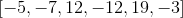Possible Answers:Correct answer:Explanation:

The range is the difference between the largest and smallest numbers.

The largest number is:The smallest number is:Subtract both numbers.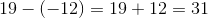The answer is:### Example Question #5 : Range

Determine the range of the numbers: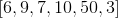Possible Answers: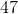Correct answer:Explanation:

The range is the difference between the largest and smallest numbers.

The largest number is 50, and the smallest number is 3.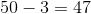The answer is:### Example Question #21 : Data Analysis And Statistics

Find the range given the following numbers: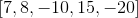Possible Answers:Correct answer:Explanation:

The range is the difference between the largest and smallest numbers.

The largest number is 15.

The smallest number is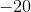.

Subtract the two numbers.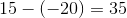The answer is:### Example Question #7 : Range

Determine the range: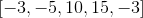Possible Answers:Correct answer:Explanation:

The range is the difference between the largest and smallest numbers.

The largest number is 15.

The smallest number is -5.

Subtract both numbers.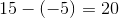The answer is.

### Example Question #1 : Standard Deviation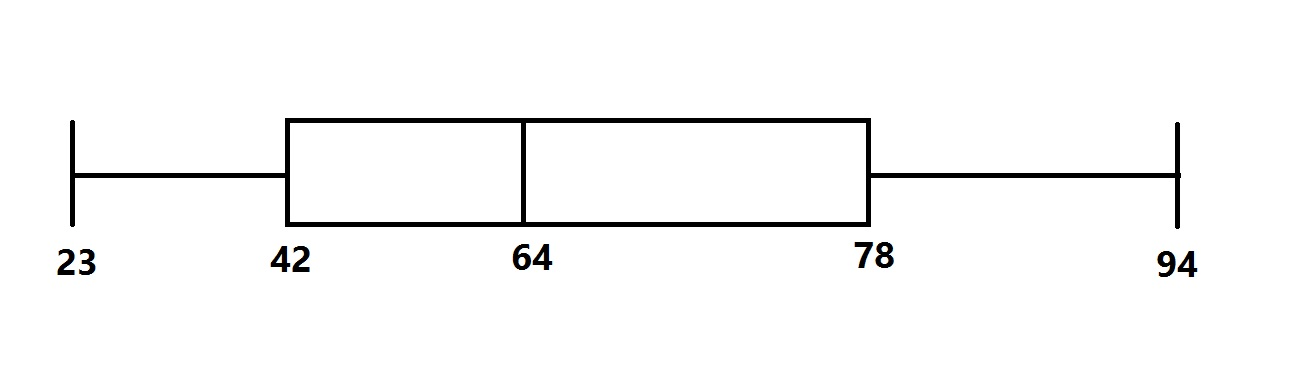The distribution of scores for a test given to a large group of students is represented by the above box-and-whisker plot.

To the nearest whole number, what was the standard deviation of the scores?

Possible Answers:Insufficient information is given to answer the question.Correct answer:

Insufficient information is given to answer the question.

Explanation:

A box-and-whisker plot gives the lowest and highest scores and the three quartiles (including the median), which depend on the relative position of the scores. The standard deviation of the scores depends on the scores themselves, which are not reflected in the diagram. The question cannot be answered from the box-and-whisker plot.

### Example Question #1 : Quartiles And Interquartile Range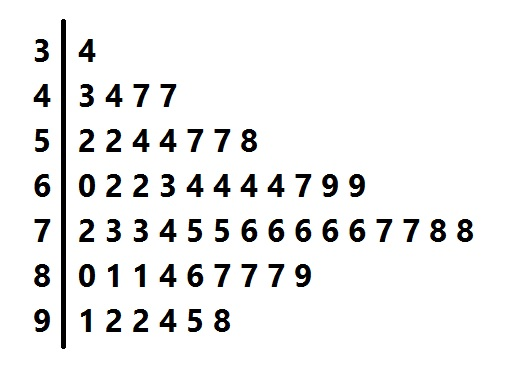Above is the stem-and-leaf display for a group of test scores. Give the interquartile range of the scores represented.

Possible Answers: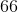Correct answer:Explanation:

The interquartile range is the difference between the third and first quartiles. To find these quartiles, first find the median of the scores. There are 53 scores represented, so look for the score in position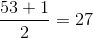.

As can be seen in this diagram, the score is 74.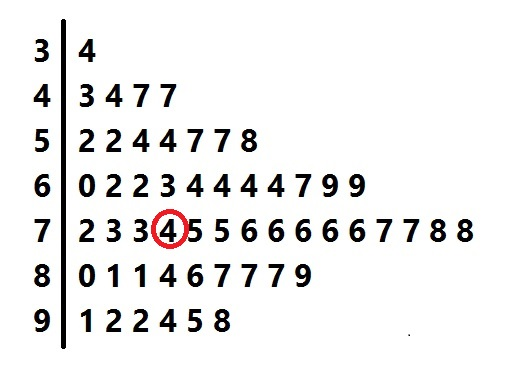The first quartile is the median of the lower half of the scores - that is, the lower 26 scores. Since 26 is even, the median is the mean of the scores in positions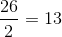and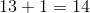.

As can be seen in this diagram, these scores are 60 and 62.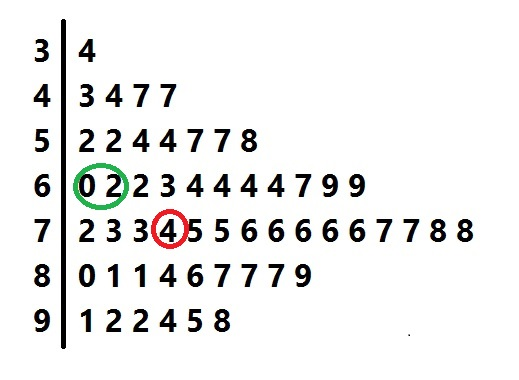The first quartile of the scores is therefore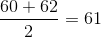The third quartile, similarly, can be found by finding the mean of the 13th and 14th elements in the top half of the scores: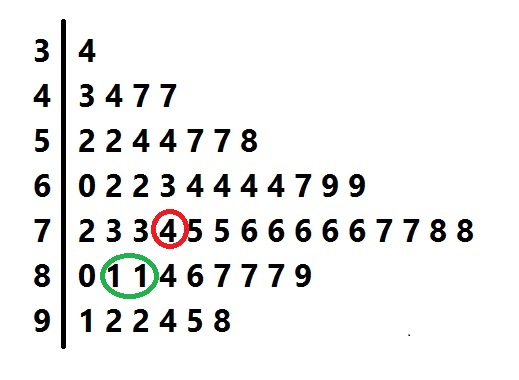As can be seen in this diagram, these scores are 81 and 81, so the third quartile is 81.

The interquartile range is the difference: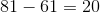### Example Question #1 : Quartiles And Interquartile RangeAbove is the stem-and-leaf display for a group of test scores. What score is at the third quartile?

Possible Answers: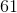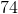Correct answer:Explanation:

To find the third quartile, first find the median of the scores. There are 53 scores represented, so look for the score in position.

As can be seen in this diagram, the score is 74.The third quartile is the median of the upper half of the scores - that is, the higher 26 scores. Since 26 is even, the median is the mean of the scores in positionsandfrom the top.As can be seen in this diagram, both of these scores are 81, so the third quartile is 81.

### Example Question #2 : Quartiles And Interquartile RangeAbove is the stem-and-leaf display for a group of test scores. What is the first quartile?

Possible Answers:Correct answer:Explanation:

To find the first quartile, first find the median of the scores. There are 53 scores represented, so, 53 being odd, look for the score in the center. This is the score in position.

As can be seen in this diagram, the score is 74.The first quartile is the median of the lower half of the scores - that is, the lower 26 scores. Since 26 is even, the median is the mean of the scores in positionsandfrom the bottom.

As can be seen in this diagram, these scores are 60 and 62.The first quartile of the scores is therefore.

### Example Question #4 : Quartiles And Interquartile Range

Consider the following numbers: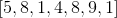.  What is the value of the first quartile?

Possible Answers:Correct answer:Explanation:

The first quartile is Q1.

Reorganize the numbers in chronological order.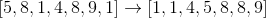The Q1 is the median of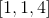, which is the three numbers left of the median of the entire set of numbers, 5.

The answer is:### Example Question #1 : Probability

Two dice, one red and one blue, are altered. The red die comes up a "1"of the time and a "2"of the time, and the blue die comes up a "1"of the time and a "2"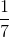of the time. What are the odds against rolling a "2", a "3", or a "4" with the two dice?

Possible Answers:

Insufficient information is given to solve the problem.

8 to 3

11 to 3

6 to 1

23 to 5

Correct answer:

Insufficient information is given to solve the problem.

Explanation:

A "2" can only be rolled on the two dice with a double "1";, a "3", with a "1-2" or "2-1", and a "4", with a "1-3", a "2-2", or a "3-1". This makes six favoriable rolls. To answer the question, we must know the probabilities that the red die will come up "1", "2", and "3"; we must also know the same for the blue die. We know the probabilities for "1" and "2" for each die, but not "3". Therefore, insufficent information is given in the problem.

### All SAT II Math II Resources